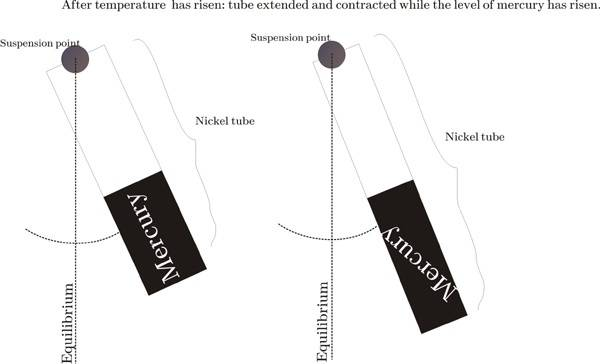# Mercury-compensated pendulum

SimonasV

## Homework Statement

Mercury-compensated pendulum

A small part of Nickel tube is fulfilled with mercury.

(Alpha) Linear coefficient of expansion (Nickel)= 1x10^-5
(Beta) Volumetric coefficient of expansion (Mercury)= 18X10^-5

FIND: What part of tube should be fullfilled, that the period of the pendulum would not depend upon temperature. And there is two different cases:
a) When centre of mass coincide with centre of Mercury in that tube.
b) Include misalignment of centres...

## Homework Equations

T=2pi(l/g)^1/2

deltaV/V=Beta*deltaT

deltaL/L=Alpha*deltaT

L=L0(1+alpha*deltaT)
V=V0(1+Beta*deltaT)

Should be more but don't know. Maybe someone could help :-)

Last edited:

Gold Member
Hello simon. Would you happen to have any form of diagrams? I am having a difficult time imagining this. What is the tube connected to? Am I to assume the tube is the bob? some more details on the setup would be nice

SimonasVcases: a) the upper level of mercury should coincide with the mass centre of whole pendulum and b) it should not coincide.

#### Attachments

Staff Emeritus
Homework Helper
Hello SimonasV,

Think about: what is "l" in the pendulum equation?

SimonasV
l=L - length of pendulum

Staff Emeritus